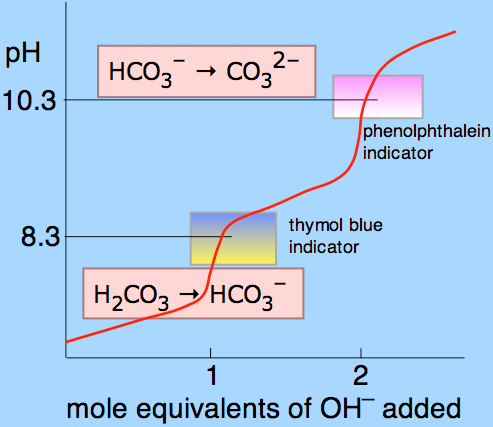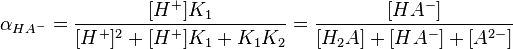## Diprotic and Polyprotic Acids

Diprotic and polyprotic acids contain multiple acidic protons that dissociate in distinct, sequential steps.

### Learning Objectives

Identify the key features that distinguish polyprotic acids from monoprotic acids.

### Key Takeaways

#### Key Points

• Polyprotic acids can lose two or more acidic protons; diprotic acids and triprotic acids are specific types of polyprotic acids that can lose two and three protons, respectively.
• Polyprotic acids display as many equivalence points in titration curves as the number of acidic protons they have; for instance, a diprotic acid would have two equivalence points, while a triprotic acid would have three equivalence points.
• For polyprotic acids, the first Ka is always the largest, followed by the second, etc.; this indicates that the protons become successively less acidic as they are lost.
• Although the tendency to lose each acidic proton decreases as subsequent ones are lost, all possible ionic species do exist in solution; to calculate their fractional concentration, one can use equations that rely on equilibrium constants and the concentration of protons in solution.

#### Key Terms

• titration: determining a substance’s concentration in a solution by slowly adding measured amounts of another substance (often with a burette) until a reaction is shown complete
• equivalence point: the point at which an added titrant is stoichiometrically equal to the number of moles in a sample’s substance; the smallest amount of titrant necessary to fully neutralize or react with the analyte
• diprotic acid: one that contains within its molecular structure two hydrogen atoms per molecule capable of dissociating
• triprotic acid: one that can donate three hydrogen ions per molecule during dissociation

As their name suggests, polyprotic acids contain more than one acidic proton. Two common examples are carbonic acid (H2CO3, which has two acidic protons and is therefore a diprotic acid) and phosphoric acid (H3PO4, which has three acidic protons and is therefore a triprotic acid).

Diprotic and polyprotic acids show unique profiles in titration experiments, where a pH versus titrant volume curve clearly shows two equivalence points for the acid; this is because the two ionizing hydrogens do not dissociate from the acid at the same time. With any polyprotic acid, the first amd most strongly acidic proton dissociates completely before the second-most acidic proton even begins to dissociate.Titration curve of carbonic acid: The titration curve of a polyprotic acid has multiple equivalence points, one for each proton. In carbonic acid’s case, the two ionizing protons each have a unique equivalence point.

### Diprotic Acids

A diprotic acid (here symbolized by H2A) can undergo one or two dissociations depending on the pH. Dissociation does not happen all at once; each dissociation step has its own Ka value, designated Ka1 and Ka2:

$\text{H}_2\text{A}(\text{aq}) \rightleftharpoons \text{H}^+(\text{aq}) + \text{HA}^-(\text{aq}) \quad\quad \text{K}_{\text{a}1}$

$\text{HA}^-(\text{aq}) \rightleftharpoons \text{H}^+(\text{aq}) + \text{A}^{2-}(\text{aq})\quad\quad \text{K}_{\text{a}2}$

The first dissociation constant is necessarily greater than the second ( i.e. Ka1 > Ka2); this is because the first proton to dissociate is always the most strongly acidic, followed in order by the next most strongly acidic proton. For example, sulfuric acid (H2SO4) can donate two protons in solution:

$\text{H}_2\text{SO}_4(\text{aq})\rightarrow \text{H}^+(\text{aq})+\text{HSO}_4^-(\text{aq})\quad\quad \text{K}_{\text{a}1}=\text{large}$

$\text{HSO}_4^-(\text{aq})\rightleftharpoons \text{H}^+(\text{aq})+\text{SO}_4^-(\text{aq})\quad\quad \text{K}_{\text{a}2}=\text{small}$

This first dissociation step of sulfuric acid will occur completely, which is why sulfuric acid is considered a strong acid; the second dissociation step is only weakly dissociating, however.

### Triprotic Acids

A triprotic acid (H3A) can undergo three dissociations and will therefore have three dissociation constants: Ka1 > Ka2 > Ka3. Take, for example the three dissociation steps of the common triprotic acid phosphoric acid:

$\text{H}_3\text{PO}_4(\text{aq})\rightarrow \text{H}^+(\text{aq})+\text{H}_2\text{PO}_4^-(\text{aq})\quad\quad \text{K}_{\text{a}1}=\text{large}$

$\text{H}_2\text{PO}_4^-(\text{aq})\rightleftharpoons \text{H}^+(\text{aq})+\text{HPO}_4^{2-}(\text{aq})\quad\quad \text{K}_{\text{a}2}=\text{small}$

$\text{HPO}_4^{2-}\rightleftharpoons \text{H}^+(\text{aq})+\text{PO}_4^{3-}(\text{aq})\quad\quad \text{K}_{\text{a}3}=\text{smallest}$

### Fractional Concentration of Conjugate Base Species

Although the subsequent loss of each hydrogen ion is less favorable, all of a polyprotic acid’s conjugate bases are present to some extent in solution. Each species’ relative level is dependent on the pH of the solution. Given the pH and the values of Ka for each dissociation step, we can calculate each species’ fractional concentration, α (alpha). The fractional concentration is defined as the concentration of a particular conjugate base of interest, divided by the sum of all species’ concentrations. For example, a generic diprotic acid will generate three species in solution: H2A, HA, and A2-, and the fractional concentration of HA, which is given by:

$\alpha=\frac{[\text{HA}^-]}{[\text{H}_2\text{A}]+[\text{HA}^-]+[\text{A}^{2-}]}$

The following formula shows how to find this fractional concentration of HA, in which pH and the acid dissociation constants for each dissociation step are known:Fractional ion calculations for polyprotic acids: The above complex equations can determine the fractional concentration of various ions from polyprotic acids.

## Calculating Equilibrium Concentrations of Polyprotic Acids

Polyprotic acids have complex equilibria due to the presence of multiple species in solution.

### Learning Objectives

Solve equilibrium problems using the appropriate approximations for weak and strong polyprotic acids.

### Key Takeaways

#### Key Points

• Polyprotic acids contain multiple acidic protons that can sequentially dissociate from the compound with unique acid dissociation constants for each proton.
• Due to the variety of possible ionic species in solution for each acid, precisely calculating the concentrations of different species at equilibrium can be very complicated.
• Certain simplifications can make the calculations easier; these simplifications vary with the specific acid and the solution conditions.

#### Key Terms

• ionic species: chemical species with a residual charge; in acid-base equilibria, the charge resulting from the loss or addition of electrons from chemical compounds
• equilibrium: the state of a reaction in which the rates of the forward and reverse reactions are the same
• polyprotic acids: an acid with multiple acidic protons

Polyprotic acids can lose more than one proton. The first proton’s dissociation may be denoted as Ka1 and the constants for successive protons’ dissociations as Ka2, etc. Common polyprotic acids include sulfuric acid (H2SO4), and phosphoric acid (H3PO4).

When determining equilibrium concentrations for different ions produced by polyprotic acids, equations can become complex to account for the various components. For a diprotic acid for instance, we can calculate the fractional dissociation (alpha) of the species HA using the following complex equation:Equation for finding the fractional dissociation of HA-: The above concentration can be used if pH is known, as well as the two acid dissociation constants for each dissociation step; oftentimes, calculations can be simplified for polyprotic acids, however.

We can simplify the problem, depending on the polyprotic acid. The following examples indicate the mathematics and simplifications for a few polyprotic acids under specific conditions.

### Diprotic Acids With a Strong First Dissociation Step

As we are already aware, sulfuric acid’s first proton is strongly acidic and dissociates completely in solution:

H2SO4 → H+ + HSO4

However, the second dissociation step is only weakly acidic:

$\text{HSO}_4^- \rightleftharpoons \text{H}^+ + \text{SO}_4^{2-}$  Ka2 = 1.20×10-2   pKa2 = 1.92

Because the first dissociation is so strong, we can assume that there is no measurable H2SO4 in the solution, and the only equilibrium calculations that need be performed deal with the second dissociation step only.

### Determining Predominant Species From pH and pKa

Phosphoric acid, H3PO4, has three dissociation steps:

$\text{H}_3\text{PO}_4 \rightleftharpoons \text{H}^+ + \text{H}_2\text{PO}_4^-$  pKa1 = 2.12

$\text{H}_2\text{PO}_4^- \rightleftharpoons \text{H}^+ + \text{HPO}_4^{2-}$   pKa2 = 7.21

$\text{HPO}_4^{2-} \rightleftharpoons \text{H}^+ + \text{PO}_4^{3-}$  pKa3 = 12.67

Thus, in an aqueous solution of phosphoric acid, there will theoretically be seven ionic and molecular species present: H3PO4, H2PO4, HPO42-, PO43-, H2O, H+, and OH.

At a pH equal to the pKa for a particular dissociation, the two forms of the dissociating species are present in equal concentrations, due to the following mathematical observation. Take for instance the second dissociation step of phosphoric acid, which has a pKa2 of 7.21:

$\text{pK}_{\text{a}2}=-\text{log}\left(\frac{[\text{H}^+][\text{HPO}_4^{2-}]}{[\text{H}_2\text{PO}_4^-]}\right)=7.21$

$\text{pH}=-\text{log}[\text{H}^+]=7.21$

By the property of logarithms, we get the following:

$\text{pH}-\text{pK}_{\text{a}2}=-\text{log}\left(\frac{[\text{HPO}_4^{2-}]}{[\text{H}_2\text{PO}_4^-]}\right)=0$

$\frac{[\text{HPO}_4^{2-}]}{[\text{H}_2\text{PO}_4^-]}=1$

Thus, when pH = pKa2, we have the ratio [HPO42-]/[H2PO4] = 1.00; in a near-neutral solution, H2PO4 and HPO42- are present in equal concentrations. Very little undissociated H3PO4 or dissociated PO43- will be found, as is determined through similar equations with their given Ka‘s.

The only phosphate species that we need to consider near pH = 7 are H2PO4 and HPO42-. Similarly, in strongly acidic solutions near pH = 3, the only species we need to consider are H3PO4 and H2PO4. As long as the pKa values of successive dissociations are separated by three or four units (as they almost always are), matters are simplified. We need only consider the equilibrium between the two predominant acid/base species, as determined by the pH of the solution.Phosphoric acid: The chemical structure of phosphoric acid indicates it has three acidic protons.

### Diprotic Acids With a Very Weak Second Dissociation Step

When a weak diprotic acid such as carbonic acid, H2CO3, dissociates, most of the protons present come from the first dissociation step:

$\text{H}_2\text{CO}_3 \rightleftharpoons \text{H}^+ + \text{HCO}_3^-$  pKa1 = 6.37

Since the second dissociation constant is smaller by four orders of magnitude (pKa2 = 10.25 is larger by four units), the contribution of hydrogen ions from the second dissociation will be only one ten-thousandth as large. Consequently, the second dissociation has a negligible effect on the total concentration of H+ in solution, and can be ignored.